Next: The Pasrec package Up: Entire functions with double recurrent steps, Pasrec package Previous: Entire functions with double recurrent steps, Pasrec package

# Introduction

During a talk about his article [Gra1] in Oberwolfach, D.W. Masser asked F. Gramain the following question : are the entire functionssolutions of the difference equations system(1)
(whereandare finite sequences of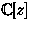with,and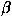being complex numbers linearly independent over) necessarily exponential polynomials, i.e. functions of the shapewith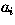inand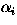in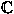for all?

In a first article [BéGra1], J.-P. Bézivin and F. Gramain gave a negative answer to this question considering the example of the function, and showed that ifbelongs to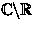and if the coefficients of one of the two equations are constant, thenis an exponential polynomial. Moreover, they proved, still assuming thatbelongs to, a serie of partial results that allowed us to conjecture that every entire solution of a system with polynomial coefficients is the quotient of an exponential polynomial by a polynomial. This conjecture has been proved with other results in a second article [BéGra2] :

Théorème  [J.-P. Bézivin- F. Gramain]
Letan entire function entière solution of the system (1) thenis the quotient of an exponential polynomial by a polynomial.

In fact, we can replace the right members of (1) by exponential polynomials. We only have to notice, as in the "Lemme 2.5" of [BéGra1], that for every complex numberand for every exponential polynomial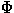, there exists a finite relation of linear dépendence overbetween the shifts ofby(we say thatis a recurrent step for the entire function-it is a definition due to D. Masser).

In the last part of [BriHab], we present an algorithm that allows to determine the entire solutions of the systèm (1).

Moreover, J.-P. Bézivin and F. Gramain proved in [BéGra2] that if we replace, in the second equation of (1), the shifts ofbyby derivatives of, an entire solutionis still the quotient of an exponential polynomial by a polynomial. The algorithm slightly modified allows again to determine the entire solutionsof the system.(2)
whereandare finite sequences ofwith,being a non zero complex number.Next: The Pasrec package Up: No Title Previous: No Title